﻿ Free Convection in a Porous Square Enclosure with Localized Sinusoidally Varying Temperature Profile on the Bottom WallPublications are Open
Access in this journal
Article Versions
Export Article
• Normal Style
• MLA Style
• APA Style
• Chicago Style
Research Article
Open Access Peer-reviewed

### Free Convection in a Porous Square Enclosure with Localized Sinusoidally Varying Temperature Profile on the Bottom Wall

Alhadj Hisseine Issaka Ali , Cheikh Mbow, Samba Dia, Mahamoud Youssouf Khayal, A.C. Beye
International Journal of Physics. 2017, 5(5), 162-170. DOI: 10.12691/ijp-5-5-4
Published online: October 23, 2017

### Abstract

This current study focuses on the simulation of free convection in square cavity filled with a porous medium considered homogenous, isotropic and saturated by a Newtonian fluid obeying the law of Darcy and the hypothesis of Boussinesq. The lower horizontal wall of the enclosure is subjected to a localized temperature varying sinusoidally with the space while the upper horizontal wall and rest of the bottom walls are insulated. The vertical walls are kept cold isotherm. In order to generalize the results, all governing equations are put into dimensionless form, discretized by the Finite Difference Method and solved by the relaxed Gauss Seidel (SUR) Algorithm. A code has been proposed in FORTRAN 95, in order to solve numerically the equations of the problem. The study parameters are the Rayleigh-Darcy number (Ra) and the amplitude (Ar) of the hot wall temperature. The effects of the Rayleigh-Darcy number, amplitude and length of source heating on the dynamic and thermal fields are investigated. On the other hand, the effects of the amplitude on the horizontal velocity distribution and the mean horizontal temperature distribution(y=0.5) were presented and discussed. It emerges from this study that the increasing of the amplitude and Rayleigh-Darcy number intensify the flow and the global heat transfer in our physical domain.

### 1. Introduction

Nowadays convection transfer in a porous medium saturated by a fluid is a paramount importance in the field of engineering and Physics. Its applications are encountered in several fields such as geothermal energy, oil recovery, solar energy storage systems, transport of pollutants into a soil and storage chemicals materials to name but a few. The problem of convection in fluid saturated porous media has been widely studied in the past decades for its applications in thermal, electronics, and engineering 1, 2, 3, 4, 5, 6, 7, 8, 9, 10, 11, 12, 13, 14, 15, 16, 17, 18, 19. The problem of natural convection in a porous cavity whose walls are maintained at different temperatures or heat fluxes is classic problem in porous media. Numerous research projects, both theorical and experimental, have been carried out on this type of cavity 1. The literature on natural Convection in porous media is abundant. An excellent review of these studies can be found in the books of Nield and Bejan 2, Kaviany 3, Vafai 4, Ingham and Pop 5 search field. However, few studies on natural convection in porous media with variable heating have been conducted throughout the world. Schaladow et al. 6 studied natural convection in the case where a cavity is subjected to a temperature varying linearly with time. Their results show that the dynamic and thermal field are very little affected by this type of boundary conditions. Saied and al. 1 studied natural convection in a porous cavity with sinusoidal heating of the lower horizontal wall, cooling of the upper horizontal wall and vertical adiabatic walls. This study showed that overall heat transfer improves with the length of the heated part and the amplitude of the temperature. Oztop et al. 7 have made a numerical study of the magneto-convection in a non-isothermal heating enclosure. They observed that the transfer increases when the amplitude of the temperature increases and decreases with the number of Hartmann. Kalabin et al. 8 have studied the convective transfer in a square inclined cavity subjected to a variable temperature on its right vertical wall. They found that the heat transfer was maximal for an inclination equal to 54 degrees and for a non-dimensional frequency f=20. Kazmierczak et al. 9analyzed the effect of a temperature varying sinusoidally over time on heat transfer and fluid flow in square cavity. They showed that the mean transfer is almost insensitive to the variation of the period. Varol et al. 10 analyzed the natural convention in a porous medium with a horizontal temperature varying sinusoidally while other walls are maintained adiabatic. They found that the transfer rate increases with the amplitude of the temperature and decreases with the aspect factor. Deng al. 11 carried out numerical study of convection in a rectangular enclosure with temperatures of the vertical walls varying sinusoidaly. They found that non-uniform heating improves heat transfer by uniform heating.

The main objective of this work is to continue to enrich this kind of problem by conducting a numerical study of natural convection in a porous square cavity saturated by a Newtonian fluid lower horizontal wall of the enclosure is subjected to a localized temperature varying sinusoidally with the space while the upper horizontal wall and rest of the bottom walls are insulated. The vertical walls are kept cold isotherm Figure 1. This study is very important because this kind of temperature variation occurs in the applications when a cylindrical heater is placed on a flat wall partially heated. The influence of the amplitude, length of heat source on the thermal and dynamic fields and the effects of amplitude on the temperature and velocity distributions of the mean horizontal plane (y = 0.5) on the other hand has been investigated for a best control of convection in our cavity.

• Figure 1. Schematic diagram of the physical model and coordinate system

### 2. Materials and Methods

2.1. Mathematical Formulation

The system studied here is a porous square cavity saturated with a Newtonian fluid. The lower horizontal wall of the enclosure is subjected to a temperature varying sinusoidally with the space while the upper horizontal wall is maintained adiabatic. The vertical walls are kept cold isotherm. The simplifying assumptions adopted are as follows:

(i) The flow is laminar and two-dimensional and follows the law of Darcy;

(ii) The porous medium saturated by the fluid is assumed to be homogeneous and in thermal equilibrium.(1)(2)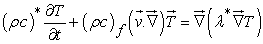(3)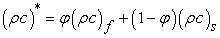(4)

Under the boundary conditions are as follows:(5)(6)

Equations (1) - (3) can be written in terms of stream function (5) - (6) by positing:(7)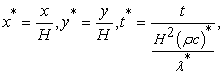(8)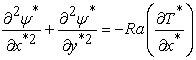(9)(10)

Where the Darcy-Rayleigh number is defined by: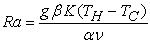(11)

With: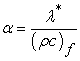(12)

The Boundary Conditions (5) - (6) become:(13a)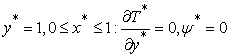(13b)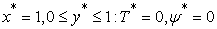(13c)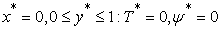(13d)
2.2. Heat Transfer

An important physical quantity in heat transfer is the Nusselt number, which is defined by the flux transferred in convection on the flux transferred in conduction state.

The local number of Nusselt at a bottom wall heated by a variable temperature is defined as follows: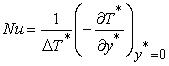(14)

The average Nusselt number is given by the following formula: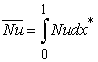(15)
2.3. Numerical Procedure

The differential equations ((9) and (10)) governing the physical situation are translated into Algebraic equations using the finite difference scheme centered and accurate on the second order. The system of dimensionless algebraic equations with boundary conditions asso- ciated (13a) - (13d) is iteratively solved by the relaxed Gauss seidel algorithm. A mesh size of 120x121 was selected. We have developed a numerical code with FORTRAN 95.

The same convergence criterion is imposed in terms of relative error for temperature and stream function. The calculation stopped when the follows inequalities were satisfied:(16)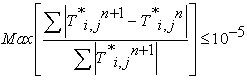(17)

### 3. Results and Discussions

3.1. Validation of the Code

A sensitivity test allowed us to choose a uniform mesh size of 120x121 and the time step used is 10-4. The results provided by the present complete code solving the problem of natural convection in porous media have been confronted with the results obtained from the work of Manole and Lage 15, Bejan 17, and Baytas and pop 12, for a stationary state of convection in a porous cavity saturated by a Newtonian fluid and whose vertical walls are active (The left vertical wall is heated by a constant temperature while the vertical wall on the right is cooled with a constant temperature) while the horizontal ones are insulated. The Table 1 compares the average Nusselt number on the left hot wall for three Darcy-Rayleigh numbers (10, 100, and 1000) with the previous work cited above. The results obtained by the present code, in the case where the side walls are active while those horizontal are maintained adiabatic, are in very good agreement with the above-mentioned authors.

3.2. Effect of Darcy-Rayleigh Number

The evolution of the thermal and dynamic field in our enclosure is shown Figure 2 for the following Rayleigh numbers: Ra = 20, 100, 500, and 1000. In each case, the fluid ascends along the vertical axis of symmetry from the middle of the hot wall and then comes into contact with the adiabatic wall which horizontally orientates its movement towards the cool isothermal vertical walls, the fluid descends along the Cold vertical isothermal walls and then goes to the center of the hot wall to complete the first cycle and start another cycle. The rights cells rotate clockwise while the left ones rotate in the opposite direction. However, there is a slight difference in flow intensity due to the spatial variation of the temperature of the hot horizontal wall. When the Rayleigh number is low (Ra = 100), the flow convection is insignificant. Therefore, heat transfer is dominated by diffusion and isotherms tend to conform to the geometry of the enclosure. The maximum steam function values for Ra = 2.101, 102, 5.102, 103 are respectively equal to: 0.8228.5.167, 19.0183, and 28.2869. It is observed that when the number of Rayleigh increases, the flow becomes more intense and is materialized by a high concentration of the streamlines in the cold walls.

3.3. Effect of the Heat Source Size

The fluid flow and heat transfer behaviors with the change of discrete heat source size are investigated by performing numerical simulations for the porous square enclosure at different discrete heat source lengths of 0.5 to 0.9 are shown in Figure 3 for a representative case of Ra=102 and Ar=0.8. It is seen that the flow fields are qualitatively identical for different heat source size for a fixed Darcy-Rayleigh number and fixed amplitude. However, quantitative results of the maximum values of the stream functions increase with increasing the length of heat source size. The maximum values of stream functions are : 4.482,4.706,4.707 for the length of heat source size equal to:0.5,0.7,0.9 respectively. In the other hand, the isotherms are affected by increasing the length of heat source size, as expected.

3.4. Amplitude Effect on the Dynamic and Thermal Fields

Figure 4 shows the temperature evolution on the mean horizontal plane (y = 0.5) for a fixed Rayleigh number (Ra = 102). The temperature on the horizontal plane on the mean plane (y = 0.5) has a Parabolic profile for Ra=100 and reaches his maximum at the point of abscissa x equal to 0.5. This maximum reached by temperature increases as the amplitude of the temperature on the horizontal wall increases. We have remarked that for Ra = 500, Ra = 1000, the temperature profile is not parabolic. The maximum reached by the temperature decreases as the Rayleigh number increase.

3.5. Amplitude Effect on the Temperature at Horizontal Plane(y=0.5)

Figure 4 shows the evolution of the temperature on the mean horizontal plane (y = 0.5) for a fixed Rayleigh number (Ra = 102). The temperature distribution on the horizontal plane (y=0.5) has a parabolic profile for Ra=100 and recaches his maximum at the point of abscissa x equal to 0.5This maximum reached by temperature inceases as the amplitude of the temperature on the horizontal wall increases.we have remarked that for Ra=500 and Ra=1000, the temperature on the horizontal, the temperature profile is not parabolic. The maximum reached by the temperature decreases as the Rayleigh number inceases.

• Figure 5. Temperature in middle horizontal plane(y=0.5) for Ra=500 and Ar=0, 0.2, 0.4, 0.6, 0.8, 1.0 at steady solution
• Figure 6. Velocity for Ra=100,500 and 1000 with Ar =0, 0.6,1 at steady solution
3.6. Amplitude Effect on the Horizontal Component of the Velocity at Horizontal Plane(Y=0.5)

Figure 6 shows the evolution of the horizontal component of the velocity on the mean horizontal plane (y = 0.5) for a fixed Rayleigh number (Ra = 102). That the amplitude of the temperature is great. In addition, there is a shift towards the interior of the cell of the extremum cell with the increase of the amplitude of the temperature (Ar). However, we have observed two local maxima for Ra = 100 while the number of Local maxima is equal to four for Ra = 500 and Ra = 1000.

3.7. Amplitude Effect on the Global Heat Transfer

Figure 7 shows the evolution of the mean Nusselt number as a function of the Rayleigh number for different amplitudes. For a fixed amplitude, the average Nusselt number increases as the number of Rayleigh increases. Similarly, for a fixed value of Rayleigh (Ra), the number of Nusselt is as important as the amplitude is high. Thus, increasing the amplitude in the range of amplitude and that of selected Rayleigh numbers improves the transfer global at bottom wall. It is also observed that the effect of the amplitude is greater when the Rayleigh-Darcy number is large.

• Figure 7. Average Nusselt number for Ar=0, 0.2, 0.4, 0.6, 0.8, 1.0 at steady solution

### 4. Conclusion

In the present paper, we have carried out a numerical study of free convection in a square cavity filled with a porous medium and saturated by an incompressible Newtonian fluid. The lower horizontal wall of the enclosure is subjected to a localized temperature varying sinusoidally with the space while the upper horizontal wall and rest of the bottom walls are insulated. The vertical walls are kept cold isotherm. The mathematical model used is that of Darcy with the Boussinesq approximation. The results produced by our calculation code were compared with some results available in the literature; a very good agreement was found between them. The results show that the overall heat transfer rate and the flow intensity increase as the Rayleigh-Darcy number increases in the selected Rayleigh range for fixed temperature amplitude. Similarly, for a fixed Rayleigh number, the overall heat transfer rate and the maximum achieved by the horizontal component of the velocity and the temperature on the mean horizontal plane (y = 0.5) increase as the amplitude of temperature increases in the chosen range of amplitudes. Finally, the results show that the flow intensity increase as the length on heat source increases for a fixed temperature amplitude and fixed Darcy-Rayleigh number. The results obtained in this study are consistent and promising in understanding the phenomenon. However, it must be recognized that the effect of the third direction on convection was not considered in this work, so a three-dimensional study could be a natural consequence of this work in order to really analyze the different Structures and aspects of free convection.

### Nomenclature

g: gravitational accelerationm.s-2

K: permeability of the porous medium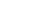m2

H: cavity Heightm

Nu: local Nusselt number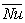: Average Nusselt number

Ra: Rayleigh-Darcy number

T: fluid temperatureK

TC: temperature of the cold wallsK

TH: temperature of the hot wallK

u, v: velocity components along x and y-axes, respectivelym.s-1

x, y: Cartesian coordinatesm

Ar: Amplitude of temperature

x*, y*: non-dimensional Cartesian coordinates

T*: non-dimensional temperature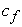: Thermal capacity of fluidJ.kg-1.K-1: Thermal capacity of porous mediaJ.kg-1.K-1: Times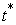: Non-dimensional time

### Greek Letters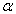: Thermal diffusivity coefficientm2.s-1: Coefficient of thermal expansionK-1: Kinematic viscositym2.s-1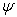: Stream functionm2.s-1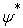: Non-dimensional stream function: Density of fluidkg.m-3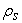: Density of porous mediakg.m-3

### Subscript

C: cold, H: hot, f: fluid, s: solid

min minimum value

max maximum value

### Superscript

* non-dimensional variables

- Average value

### Acknowledgements

We would like to express our grateful thanks and gratitude to ours Professors AC.Beye, M.Y.Khayal and C.Mbow for providing us the opportunity to carry out this research work.

### References

  Saied, N.H., 2005. Natural convection in a square porous cavity with an oscillating wall temperature. Arabian J.Sci.Eng. 31: 35-46. In article View Article  Nield, DA and A.Bejan, 2006. Convection in porous Media, 3rd Edn. Springer Science and Business Media, New York, pp. 640. In article View Article  M. Kaviany (1995). "Principles of Heat Transfer in porous Media" Second. In article View Article  Vafai, K. 2005. Handbook of porous Media, 2nd Edition, CRC Press, New York, 784. In article View Article  Ingham, D.B and Pop., I. (2005). Transport Phenomena in Porous Media III. Elsevier, Oxford, 450. In article View Article  Schaladaw, S., Patterson, J.C and Street, R.L, (1989). Transient Flow in a side-Heated Cavity at high Rayleigh number: a numerical study. Journal of Fluid Mechanics, 200, 121-148. In article View Article  H.F. Oztop, M Oztop and Y. varol, Numerical simulation of magneto-hydrodynamic Buoyancy-Induced Flow in a non-isothermally Heated Square Enclosure, Com Nonlinear Sci. Numer., simul., vol.14, pp. 770-778, 2009. In article View Article  E.V. Kalabin, M.V. Kanashina, and P.T. Zubkov, Natural-convective Heat Transfer in a square cavity with Time-Varying side-wall Temperature, Numer. Heat Transfer A, Vol. 47, pp. 621-631, 2005. In article View Article  Kazmierczak and Chinoda, Z., (1992). Buoyancy-Driven Flow in an enclosure with time periodic Boundary Conditions. International Journal of Heat and Mass Transfer, 35, 1507-1518. In article View Article  Varol, Y., Oztop, H.F., and Pop, I., Numerical Analysis of Natural convection for Porous Rectangular Enclosure with sinusoidal Varying Temperature Profile on the Bottom wall, International Communication in Heat and mass Transfer,Vol.35, 2008, pp. 56,64. In article View Article  Q.H Deng and J.J Chang, Natural Convection in a rectangular Enclosure with sinusoidal Temperature Distribution on Both Side walls, Numer.Heat Transfer A, vol.54. pp. 507-524, 2008. In article View Article  Baytas, A.C and I. Pop, (2002). Free convection in a square porous cavity using a thermal no equilibrium model. Int. J. Thermal Sci., 41, 861-870. In article View Article  Kimura, S.K, Vynnycky.M and Alavyoon Unicellular natural circulation in a shallow horizontal porous layer heated from below by a constant flux, Fluid Mech. 294, 231-257. In article View Article  Darcy, H.P.C. Les fontaines publiques de la ville de Dijon (Victor, Dalmont, paris 1856). In article View Article  Moya, S.L and Ramos, 1987.Numerical study of a natural convection in tilted rectangular porous material.Int.J hem Mass T, 30: 741-756. In article View Article  Sheremet, M.A. and I. Pop and Ramos, 2014. Natural convection in square porous cavity with sinusoidal temperature distributions in both side walls filled with a Nanofluid: Buongiorno’s mathematical mdel. Transp. Porous Med., 105: 411-429. In article View Article  Bejan, A., (1979). On the boundary layer regime in a vertical enclosure filled with a porous medium. Lett. Heat Mass Transfer, 6, 93-102. In article View Article  Ali, A.H.I., Dia, S., Khayal, M.Y., Mbow, C. And Beye, A.C. (2017). Natural Study of Natural Convection in a Porous Square Enclosure with sinusoidally varying Temperature Profile on the Bottom wall. Open Journal of Fluid Dynamics, 7, 263-275. In article View Article  Saha, Suvash, Molla, Md. Mamum &. Khan, M. A.I (2012). Natural convection flow in a porous enclosure with localized heating from below. JP Journal of Heat and Mass Transfer, 6(1), pp.1-16. In article View Article

Published with license by Science and Education Publishing, Copyright © 2017 Alhadj Hisseine Issaka Ali, Cheikh Mbow, Samba Dia, Mahamoud Youssouf Khayal and A.C. BeyeThis work is licensed under a Creative Commons Attribution 4.0 International License. To view a copy of this license, visit http://creativecommons.org/licenses/by/4.0/